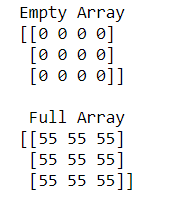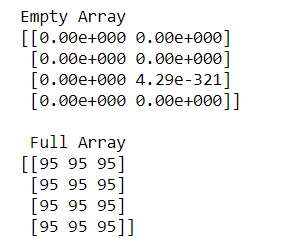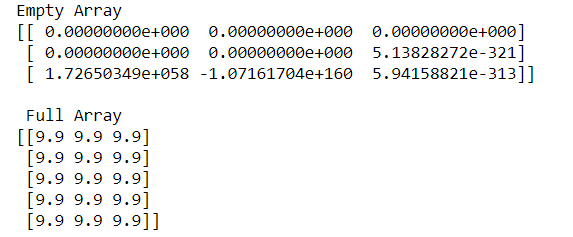# How to create an empty and a full NumPy array?

• Difficulty Level : Easy
• Last Updated : 01 Oct, 2020

Sometimes there is a need to create an empty and full array simultaneously for a particular question. In this situation, we have two functions named as numpy.empty() and numpy.full() to create an empty and full arrays.

Syntax:

```numpy.full(shape, fill_value, dtype = None, order = ‘C’)
numpy.empty(shape, dtype = float, order = ‘C’)
```

Example 1:

## Python3

 `# python program to create``# Empty and Full Numpy arrays`` ` `import` `numpy as np`` ` ` ` `# Create an empty array``empa ``=` `np.empty((``3``, ``4``), dtype``=``int``)``print``(``"Empty Array"``)``print``(empa)`` ` `# Create a full array``flla ``=` `np.full([``3``, ``3``], ``55``, dtype``=``int``)``print``(``"\n Full Array"``)``print``(flla)`

Output:In the above example, we create an empty array of 3X4 and full array of 3X3 of INTEGER type.

Example 2:

## Python3

 `# python program to create``# Empty and Full Numpy arrays`` ` `import` `numpy as np`` ` ` ` `# Create an empty array``empa ``=` `np.empty([``4``, ``2``])``print``(``"Empty Array"``)``print``(empa)`` ` `# Create a full array``flla ``=` `np.full([``4``, ``3``], ``95``)``print``(``"\n Full Array"``)``print``(flla)`

Output:In the above example, we create an empty array of 4X2 and full array of 4X3 of INTEGER and FLOAT type.

Example 3:

## Python3

 `# python program to create ``# Empty and Full Numpy arrays`` ` `import` `numpy as np`` ` `# Create an empty array``empa ``=`  `np.empty([``3``,``3``]) ``print``(``"Empty Array"``)``print``(empa)`` ` `# Create a full array``flla ``=` `np.full([``5``,``3``], ``9.9``)``print``(``"\n Full Array"``)``print``(flla)`

Output:In the above example, we create an empty array of 3X3 and full array of 5X3 of FLOAT type.

My Personal Notes arrow_drop_up